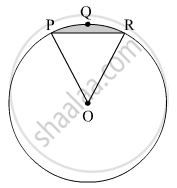Advertisement Remove all ads

# In the Given Figure, O is the Centre of the Circle. M ( Arc Pqr) = 60° Op = 10 Cm. Find the Area of the Shaded Region.( π = 3.14, √ 3 = 1.73) - Geometry

Advertisement Remove all ads
Advertisement Remove all ads
Advertisement Remove all ads
Sum

In the given figure, O is the centre of the circle. m ( arc PQR) = 60° OP = 10 cm. Find the area of the shaded region.( $\pi$= 3.14, $\sqrt{3}$= 1.73)Advertisement Remove all ads

#### Solution

Radius of the circle, r = 10 cm
m(arc PQR) = ∠POR = θ = 60º
∴ Area of the shaded region = Area of segment PQR

$= r^2 \left( \frac{\pi\theta}{360°} - \frac{\sin\theta}{2} \right)$
$= \left( 10 \right)^2 \left( \frac{3 . 14 \times 60°}{360°} - \frac{\sin60°}{2} \right)$
$= 100 \times \left( \frac{3 . 14}{6} - \frac{\sqrt{3}}{4} \right)$
$= \frac{314}{6} - \frac{173}{4} \left( \sqrt{3} = 1 . 73 \right)$
$= 52 . 33 - 43 . 25$
$= 9 . 08 {cm}^2$

Thus, the area of the shaded region is 9.08 cm2.

Concept: Areas of Sector and Segment of a Circle
Is there an error in this question or solution?

#### APPEARS IN

Balbharati Mathematics 2 Geometry 10th Standard SSC Maharashtra State Board
Chapter 7 Mensuration
Practice set 7.4 | Q 2 | Page 159
Advertisement Remove all ads
Share
Notifications

View all notifications

Forgot password?